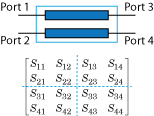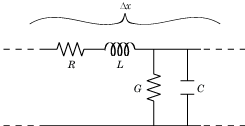# rlgc2s

Convert RLGC transmission line parameters to S-parameters

## Syntax

``s_params = rlgc2s(R,L,G,C,length,freq)``
``s_params = rlgc2s(___,z0)``

## Description

````s_params = rlgc2s(R,L,G,C,length,freq)` transforms RLGC transmission line parameter data into S-parameters with a reference impedance of 50 Ω.```

example

````s_params = rlgc2s(___,z0)` transforms RLGC transmission line parameter data into S-parameters with a reference impedance of `z0`. Use this option with the input arguments in the previous syntax.```

## Examples

collapse all

Define the variables for a transmission line.

```length = 1e-3; freq = 1e9; z0 = 50; R = 50; L = 1e-9; G = .01; C = 1e-12;```

Calculate the s-parameters.

`s_params = rlgc2s(R,L,G,C,length,freq,z0)`
```s_params = 2×2 complex 0.0002 - 0.0001i 0.9993 - 0.0002i 0.9993 - 0.0002i 0.0002 - 0.0001i ```

## Input Arguments

collapse all

Resistance matrix, specified as an N-by-N-by-M array of distributed resistances, in units of Ω/m. The N-by-N matrices must be real symmetric, the diagonal terms must be nonnegative, and the off-diagonal terms must be nonnegative.

Inductance matrix, specified as an N-by-N-by-M array of distributed inductances, in units of H/m. The N-by-N matrices must be real symmetric, the diagonal terms must be positive, and the off-diagonal terms must be nonnegative.

Conductance Matrix, specified as an N-by-N-by-M array of distributed conductances, in units of S/m. The N-by-N matrices must be real symmetric, the diagonal terms must be nonnegative, and the off-diagonal terms must be nonpositive.

Capacitance matrix, specified as an N-by-N-by-M array of distributed capacitances, in units of F/m. The matrices must be real symmetric, the diagonal terms must be positive, and the off-diagonal terms must be nonpositive.

Length of transmission line, specified as a scalar in meters.

Frequency, Specified as a vector of M frequencies over which the transmission line parameters are defined.

Reference impedance of N-port S-Parameters, specified as positive real scalar in ohms.

## Output Arguments

collapse all

S-parameters, returned as a 2N-by-2N-by-M array of complex numbers. The following figure describes the port ordering convention of the output.This port ordering convention assumes that:

• Each 2N-by-2N matrix consists of N input terminals and N output terminals.

• The first N ports (1 through N) of the S-parameter matrix are input ports.

• The last N ports (N + 1 through 2N) are output ports.

To reorder ports after using this function, use the `snp2smp` function.

collapse all

### RLCG Transmission Line Model

The following figure illustrates the RLGC transmission line model.The representation consists of:

• The distributed resistance, R, of the conductors, represented by a series resistor.

• The distributed inductance, L, represented by a series inductor.

• The distributed conductance, G,

• The distributed capacitance, C, between the two conductors, represented by a shunt capacitor.

RLGC component units are all per unit length Δx.

 Bhatti, A. A. “A Computer Based Method for Computing the N-Dimensional Generalized ABCD Parameter Matrices of N-Dimensional Systems with Distributed Parameters.”  Proceedings. The Twenty-Second Southeastern Symposium on System Theory, IEEE Comput. Soc. Press, 1990, pp. 590–93. DOI.org (Crossref), doi:10.1109/SSST.1990.138213.